/

### The Cauchy distribution

 Distribution name Cauchy distribution Common notation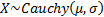Parameters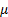= location parameter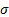= scale parameter (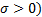Domain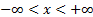Probability density function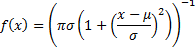Cumulative distribution function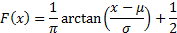Mean Does not exist Variance Does not exist Skewness Does not exist (Excess) kurtosis Does not exist Characteristic function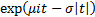Other comments The quantile function of the Cauchy distribution is: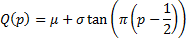Its median is thus.   The Cauchy distribution is a special case of the stable (more precisely the sum stable) distribution family.   The special case of the Cauchy distribution when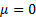and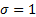is called the standard Cauchy distribution. It coincides with the Student’s t distribution with one degree of freedom. It has a probability density function of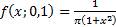.   If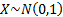and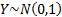are independent random variables then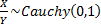and this can be used to generate random variates.   The Cauchy distribution is also known as the Cauchy-Lorentz or Lorentz distribution (especially amongst physicists).

Nematrian web functions

Functions relating to the above distribution may be accessed via the Nematrian web function library by using a DistributionName of “cauchy”. For details of other supported probability distributions see here.